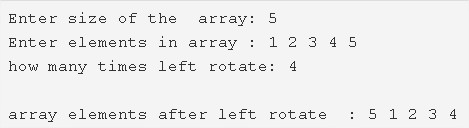# C Program To Left Rotate An Array | C Programs

C program to left rotate an array – In this article, we will brief in on the various means to left rotate an array in C programming.

Suitable examples and sample programs have also been added so that you can understand the whole thing very clearly. The compiler has also been added with which you can execute it yourself.

The means used in this piece are as follows:

• Using Standard Method
• Using Function

An array, as we all know, is a sequential order of a bunch of elements in a horizontal fashion. These elements’ position is denoted by specific entities known as pointers. Arrays are one of the most essential parts of C programming.As in the example given above, firstly, we have to enter the size of the array.

In this case, the size of the array specified is 5.

Then we have to enter the elements in the array.

The elements here are as follows:

1 2 3 4 5

Later, we have to specify the number of times we have to left rotate. The number cannot be greater than the number of elements.

Thus, by rotating the array 4 times, we get the new array:

5 1 2 3 4

Hence, doing the same in C programming is as follows:

## Using Standard Method

1. Store the array size into the variable n.

2) Read the entered array and store the elements in the array a[].

3) Read the k value, which represents how many numbers of times left rotate the given array.

4) Rotate the array to left for k times as follows, for loop iterates from i=0 to i<k

a) Assign starting element to temp.

b) Inner for loop iterates from j=0 to j<n-1

move a[j+1] to a[j].Repeat until j<n-1.

c) Assign temp to a[n-1]. Here we are assigning the first element to the last index.

Repeat these three steps until i<k.

5) After all iterations of i, print the array which is left rotated.

Output:

### Using Function

1. The main() calls the leftrotate() function, passing array,size of the array, k as arguments.

2) The leftrotate() function rotates the array to left for k times as follows,

for loop iterates from i=0 to i<k

a) assign the first element to temp,

b) Inner for loop iterates from j=0 to j<n-1

move the the element a[j+1] to a[j].Repeat this step until j<n-1.

c) Assign temp to a[n-1].

Repeat these three steps until i<k.

3) After all iterations of i, the main() call the print() to print the left rotated array elements.

Output:

x

## C Program To Print Number Of Days In A Month | Java Tutoring

C program to input the month number and print the number of days in that ...